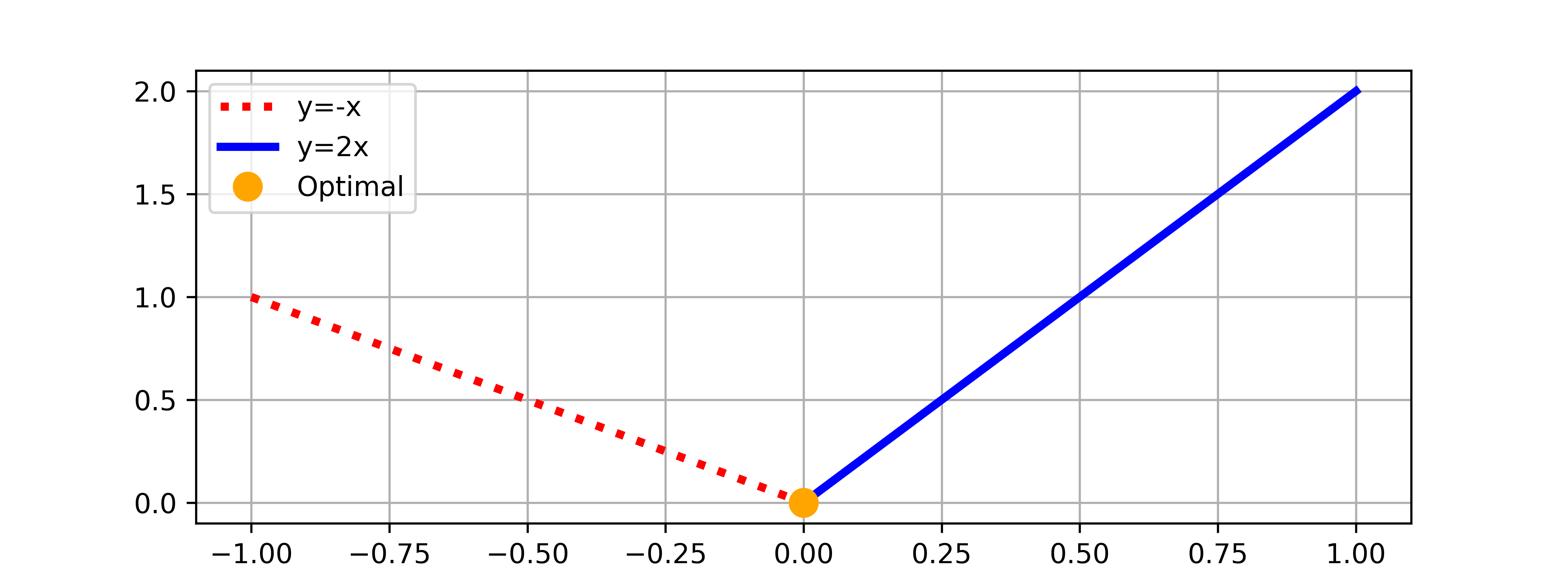## Optimize with Conditional Statements

Conditional statements (IF ELSE) require special treatment to be used in gradient-based optimization. Two popular methods are Mathematical Programs with Complementarity Constraints (MPCCs) and binary (0 or 1) switching variables. Consider the simple example where the variable y is minimumized by changing the value of x.import matplotlib.pyplot as plt
import numpy as np

x1 = np.linspace(-1,0)
x2 = np.linspace(0,1)
y1 = -x1
y2 = 2*x2

plt.figure(figsize=(8,3))
plt.plot(x1,y1,'r:',lw=3,label='y=-x')
plt.plot(x2,y2,'b-',lw=3,label='y=2x')
plt.plot(,,'o',color='orange',markersize=10,label='Optimal')
plt.grid(); plt.legend()
plt.savefig('conditional.png',dpi=600)
plt.show()

Incorrect

A common error with conditional statements is to express the condition as a typical if, else construct in Python.

from gekko import GEKKO
m = GEKKO()
x,y = m.Array(m.Var,2)
if x<=0:
y = -1.0 * x
else:
y =  2.0 * x
m.Minimize(y)
m.solve()

This form is difficult for a gradient-based optimizer because the transition between the equations y=-x / y=2*x is a switching point at zero. The gradient-based solvers are not designed for problems with non-continuous first or second derivatives. The Jacobian (1st derivatives) and Hessian (2nd derivatives) are gradients of the equations and Lagrangian function. Solvers need these to be continuous to efficiently find a solution.

Gekko Functions for Conditional Statements

There are at two methods in Gekko to provide continuous gradients for the solvers.

y = m.if2(x,x<0,x>=0)
y = m.if3(x,x<0,x>=0)

if2 (MPCC) Function

The if2 function uses a Mathematical Program with Complementarity Constraint (MPCC). The additional variables are two slack variables s_1 and s_2 and a switching variable b.

\begin{align}\min_{b,s_1,s_2,x,y} \quad y&\\s.t. \quad x&=s_1 - s_2 \\ s_1&\ge0 \\ s_2&\ge0 \\ s_1 s_2&\le0 \\ 0&=s_1\left(1-b\right)+s_2\left(b\right) \\y&=(1-b)(-x) + b(2x)\end{align}

The gradients are continuous and the complementarity constraint is an efficient way to solve the problem without using integer variables.

from gekko import GEKKO
m = GEKKO()
x = m.Var()
y = m.if2(x,-x,2*x)
m.Minimize(y)
m.solve()
print(x.value,y.value)

This form sometimes has issues if the solution is at the switching condition because it is a saddle point. This can cause the solver to fail to find a solution or report a successful solution when the conditions are not met.

if3 (Binary Variable) Function

An alternative method is a binary switching variable (b) with the if3 function in Gekko.

\begin{align}\min_{b,x,y} \quad y& \\ \mathrm{s.t.} \quad 0 &\ge(1-b) x \\ 0 &\ge b (-x) \\ y&=(1-b)(-x) + b(2x)\end{align}

Gekko automates the creation of the additional equations and binary variable with the function call. The problem is solved with a Mixed Integer programming solver such as APOPT (m.options.SOLVER=1).

from gekko import GEKKO
m = GEKKO()
x = m.Var()
y = m.if3(x,-x,2*x)
m.Minimize(y)
m.options.SOLVER = 1
m.solve()
print(x.value,y.value)

Binary (0 or 1) variables are frequently used in optimization. Examples of binary variables include On/Off state or True/False as a 1 or 0 binary value. Integer Programming is a type of optimization problem where the variables are restricted to discrete whole number values such as 0/1 binary values. A Mixed-Integer Programming problem is when some of the variables are continuous and some are discrete. Mixed-Integer Nonlinear Programming (MINLP) also includes nonlinear equations and requires specialized MINLP solvers. At first glance it might seem solving a binary variable problem would be easier than a continuous problem. This is not the case, however, because it is challenging to explore all the different combinations that occurs in all but the smallest problems. For example if 30 variables can each take 2 values, there are 2^30 (>1 billion) possibilities. Even with the fastest computer, it may take a long time to evaluate all of these combinations. There are numerical solvers in Gekko such as APOPT that use branch and bound to efficiently solve problems with binary, integer, or discrete variables.